Parenting

# 5 math hacks your child will never learn in school

307 views

 1 Introduction 2 5 math hacks your child 3 Conclusion 4 About Cuemath 5 Frequently Asked Questions (FAQs) 6 External References

## Introduction

Hacks are like magic, especially when it comes to math. These days calculators and online resources can provide the answers to almost every mathematical problem, no matter how complex.

These math hacks will help you solve tricky problems and show you that math doesn’t always have to be complicated. These are the shortcuts that your teachers do not teach you at school.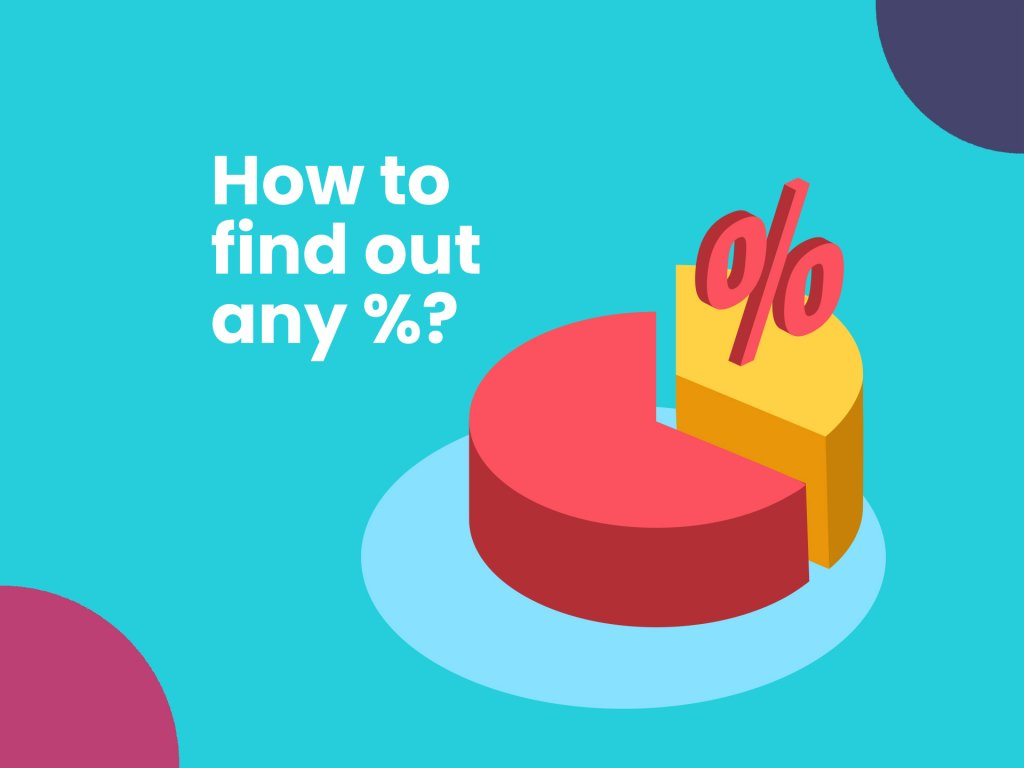### 5 math hacks your child will never learn in school-PDF

Schools teach your child the general typical way of reaching a solution. Here are 5 hacks your kids will most definitely not learn at school. Here is a downloadable PDF to explore more.

## 5 math hacks your child

### Math hack #1: How to calculate the value of any percentage without a calculator?

Percentage calculations don’t have to be complicated. There is an easy way to calculate the value of any percentage without a calculator.

When looking for the percentage value of a number, say 40% of 300.

Simply drop the one’s digit of the number (in this case 300 becomes 30 and 40 becomes 4), and multiply what remains (for this case we get 4×30 which gives us 120)

So 40% of 300= 120.

Why don’t you try 20% of 150 using this hack?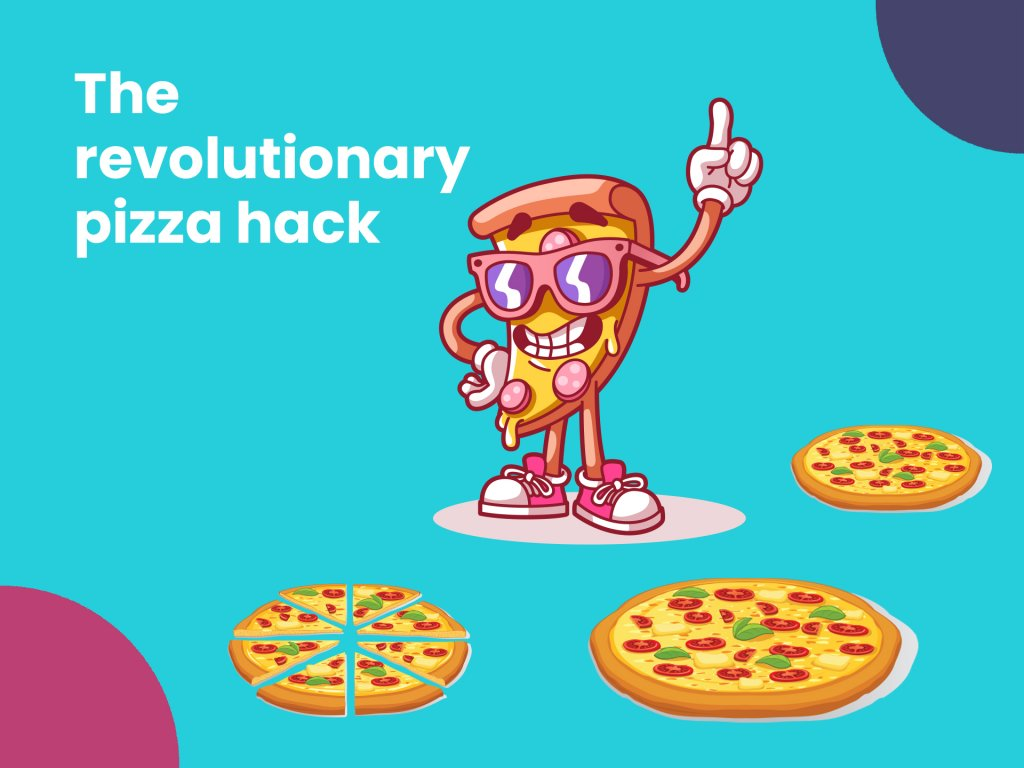### Math hack #2: The revolutionary pizza hack that will give you more pizza.

When you’re out with your family for some pizza time, chances are you ordered 2, 12-inch pizzas because logically 2 pizzas are more than one, right?

Well, that’s not entirely true.

If you calculate the area of an 18-inch pizza it actually sums up to: Area= 𝜋 (18/2)^2   = 254 in^2

While the area of two 12-inch pizza’s sums up to:  Area= 2𝜋 (12/2) ^2  = 226 in^2

So logically you’re paying more for LESS pizza!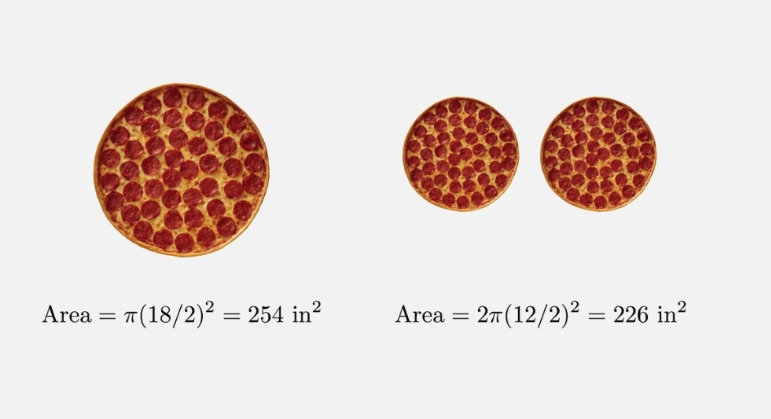### Math hack #3: Find out any day of the week, on any date without using a calendar.

This hack may sound complicated but is actually pretty fun and simple once you get used to it. Would you be able to find out which day it was on October 4, 2000, without looking through the calendar?

To work out the days of the week, we need to convert the days of the week and the months into numbers. This hack involves an easy to remember mnemonics, such as the one in this table.

Similarly the mnemonics for months in this table range from 0 to 6.

Now that you know how to calculate the day and the month, let’s move on to the years. There are two things you need to remember when calculating the years:

1.     If the year you’re calculating is a leap year, everything jumps forward by 2 days.
2.     Normal years progresses as 2000, 2001, 2002..and so on.

This table will help you understand the mnemonic to the leap years.

So what day is Oct 4, 2000?

The formula you need to use here is: Month Code + Date + Year Code = Day of Week Code.

So October according to the table is: 6

And 2000 is: 0

The date as we already know is: 4

So we have: 6+4+0= 10

But we don’t have any day in the week for that number. If you look on a calendar, the 10th of any month will always fall on the same day of the week as the 3rd, because the 3rd is 7 days earlier. So, with the result, or any number in the formula, we can subtract 7 to reduce the answer.

Therefore, 10-7= 3

So, October 4, 2000, was a Wednesday!

Go ahead, check it on the calendar.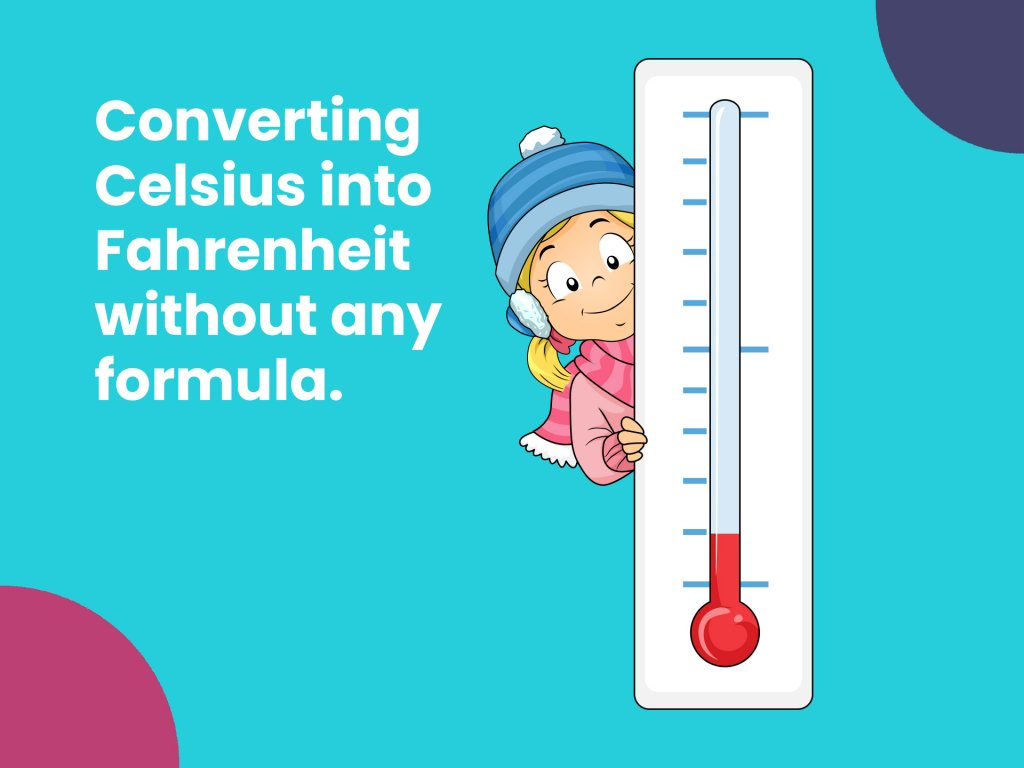### Math hack #4: Converting temperatures with ease

We’ve all learned how to convert temperatures from Celsius to Fahrenheit using this formula at school: (°C × 9/5) + 32 =°F

However, there is an easier way to convert temperatures. One that doesn’t require you to memorize a formula.
To convert Celsius (C) to Fahrenheit (F) simply multiply the temperature by 2 and add 30 to it.

Eg. If you want to convert 22oC to Fahrenheit: (22×2)+30, which roughly equates to 74oF.

To convert Fahrenheit to Celsius, simply follow the opposite.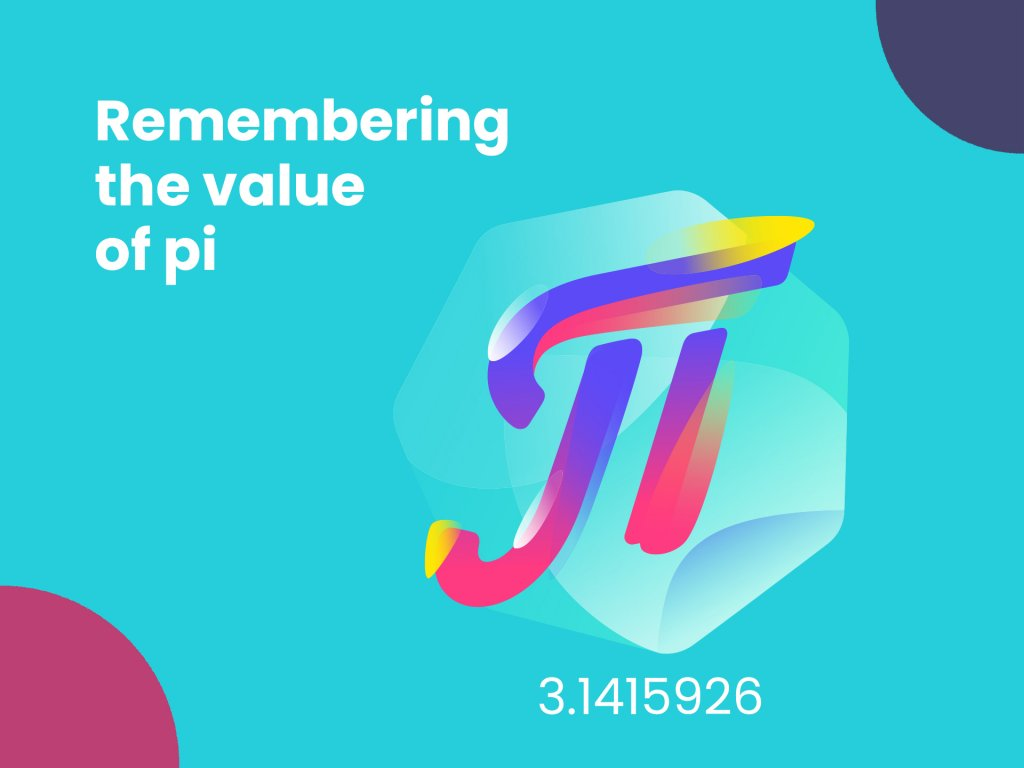### Math hack #5: Remembering the first 7 digits of the value of pi

We’ve mostly almost always used the value of pi until the first 3 digits of it, i.e 3.14. But in case you ever need to narrow down your end result to an even more exact number, there is an easier way to remember more than the first 3 digits of pi.

Just remember the sentence ‘May, I have a large container of coffee. Counting the number of letters in each word of the sentence will give you the value of the first 7 digits of pi.

May: 3, I:1, have:4, a:1, large:5, container:9, of:2, coffee:6, which is 3.1415926.

Cuemath is a beyond school math learning program that helps your child understand the WHY behind the WHAT of various math concepts through fun puzzles and math activities built to strengthen their math foundation and give them an edge over their peers.

## Conclusion

Cuemath Leap is a program designed to help young minds develop a strong mathematical foundation and a problem-solving attitude.

We emphasize Cueing the way, which makes learning fast and makes learning last. Math is a path to make the next generation of problem solvers, and we want to make sure that no child is left behind.

With strong mathematical skills, we believe one has the power to bring innovative ideas to life and to make the world a better place.

Cuemath, a student-friendly mathematics platform, conducts regular Online Live Classes for academics and skill-development, and their Mental Math App, on both iOS and Android, is a one-stop solution for kids to develop multiple skills.

## What is Cuemath?

Cuemath Leap is a beyond-school excellence program. Developed by IIT and Cambridge graduates, it aims to change the way math is taught to students. The curriculum is designed to make math fun and interesting. We cover all the math concepts from all boards- CBSE, ICSE, State Boards, and International boards.

## How to become a teacher in Cuemath?

First, one has to complete an online job application form. Shortlisted applicants need to register for training by paying the fee for an electronic writing pad. Then the applicant has to go through the training courses and pass the necessary assessment tests to get certified. No prior teaching experience is required.

## How Cuemath works?

Cuemath has programs for math and coding for students of grades 1-10. Students can request a demo session to check out the Cuemath classroom and learning experience. Once enrolled, students have live interactive online classes personalized to their needs. Proper attention is given to each student as the batch size is small, and the doubts get cleared instantly.